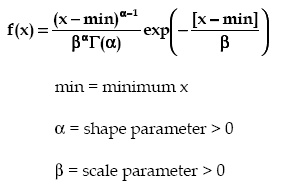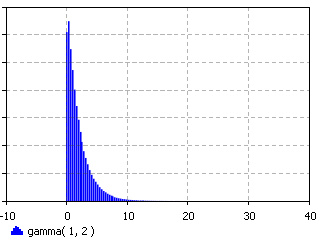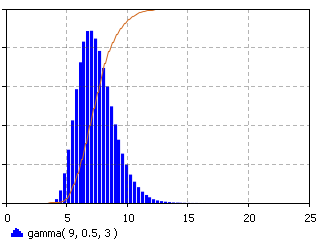AnyLogic

# gammaThe Gamma distribution is a continuous distribution bounded at the lower side. It has three distinct regions. For alpha=1, beta=1/lambda the Gamma distribution reduces to the exponential (lambda) distribution, starting at a finite value at minimum x and decreasing monotonically thereafter. For alpha < 1, the Gamma distribution tends to infinity at minimum x and decreases monotonically for increasing x. For alpha > 1, the Gamma distribution is 0 at minimum x, peaks at a value that depends on both alpha and beta, decreasing monotonically thereafter. If alpha is restricted to positive integers, the Gamma distribution is reduced to the Erlang distribution.

The Gamma distribution also reduces to the Chi Squared distribution for min = 0, beta = 2, and alpha = nμ/2. It can then be viewed as the distribution of the sum of squares of independent unit normal variables, with nµ degrees of freedom and is used in many statistical tests.

The Gamma distribution can also be used to approximate the Normal distribution, for large alpha, while maintaining its strictly positive values of x [actually (x-min)].

The Gamma distribution has been used to represent lifetimes, lead times, personal income data, a population about a stable equilibrium, interarrival times, and service times. In particular, it can represent lifetime with redundancy.

Examples of each of the regions of the Gamma distribution are shown above. Note the peak of the distribution moving away from the minimum value for increasing alpha, but with a much broader distribution.

#### Examples### gamma(double alpha, double beta, double min)

Description
Generates a sample of the Gamma distribution.
Parameters
Name Type of value Description
alpha double The shape parameter > 0.
beta double The scale parameter > 0.
min double The minimum x value.
Result
Type Description
double The generated sample.

### gamma(double alpha, double beta)

Description
Generates a sample of the Gamma distribution with min set to 0. Is equivalent to gamma(alpha, beta, 0).
Parameters
Name Type of value Description
alpha double The shape parameter > 0
beta double The scale parameter > 0.
Result
Type Description
double the generated sample

### gamma(double alpha, double beta, double min, java.util.Random r)

Description
Generates a sample of the Gamma distribution using the specified random number generator.
Parameters
Name Type of value Description
alpha double The shape parameter > 0.
beta double The scale parameter > 0.
min double The minimum x value.
r java.util.Random The random number generator.
Result
Type Description
double The generated sample.

This document includes content from the “Stat::Fit User’s Manual”. Copyright 2016 Geer Mountain Software Corp.